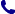• Kontakt
• Newsletter Zapisz się po informacje o nowościach i specjalnych ofertach. Administrator danych: ABE-IPS sp. z o.o.
Wybierz interesujące Cię kategorie
Wyrażam zgodę na przetwarzanie moich danych osobowych w celach marketingowych, tym samym akceptuję Regulamin Newslettera i Politykę prywatności.
Wyrażam zgodę na przesyłanie informacji handlowych drogą elektroniczną.
Wypisz się
• Klienci instytucjonalni
• Dostawa
• O nas
Dostęp on-line

# Książki

0.00 PLN
Schowek (0)
 Schowek jest pusty

## Nonlinear Evolution and Difference Equations of Monotone Type in Hilbert Spaces

 Autorzy Wydawnictwo Apple Academic Press Inc. Data wydania 21/03/2019 Liczba stron 240 Forma publikacji książka w twardej oprawie Poziom zaawansowania Literatura popularna ISBN 9781482228182 Kategorie Analizy funkcjonalne i przekształcenia, Rachunek różniczkowy i formuły
578.78 PLN (z VAT)
\$146.26 / €130.51 / £116.84 /
Produkt na zamówienie
Dostawa 5-6 tygodni
Ilość
Do schowka

## Opis książki

This book is devoted to the study of nonlinear evolution and difference equations of first and second order governed by a maximal monotone operator. This class of abstract evolution equations contains not only a class of ordinary differential equations, but also unify some important partial differential equations, such as the heat equation, wave equation, Schrodinger equation, etc. In addition to their applications in ordinary and partial differential equations, this class of evolution equations and their discrete version of difference equations have found many applications in optimization. In recent years, extensive studies have been conducted in the existence and asymptotic behaviour of solutions to this class of evolution and difference equations, including some of the authors works. This book contains a collection of such works, and its applications. Key selling features: Discusses in detail the study of non-linear evolution and difference equations governed by maximal monotone operator Information is provided in a clear and simple manner, making it accessible to graduate students and scientists with little or no background in the subject material Includes a vast collection of the authors' own work in the field and their applications, as well as research from other experts in this area of study

Nonlinear Evolution and Difference Equations of Monotone Type in Hilbert Spaces

## Spis treści

PART I. PRELIMINARIES

Preliminaries of Functional Analysis

Introduction to Hilbert Spaces

Weak Topology and Weak Convergence

Reexive Banach Spaces

Distributions and Sobolev Spaces

Convex Analysis and Subdifferential Operators

Introduction

Convex Sets and Convex Functions

Continuity of Convex Functions

Minimization Properties

Fenchel Subdifferential

The Fenchel Conjugate

Maximal Monotone Operators

Introduction

Monotone Operators

Maximal Monotonicity

Resolvent and Yosida Approximation

Canonical Extension

PART II - EVOLUTION EQUATIONS OF MONOTONE TYPE

First Order Evolution Equations

Introduction

Existence and Uniqueness of Solutions

Periodic Forcing

Nonexpansive Semigroup Generated by a Maximal Monotone Operator

Ergodic Theorems for Nonexpansive Sequences and Curves

Weak Convergence of Solutions and Means

Almost Orbits

Sub-differential and Non-expansive Cases

Strong Ergodic Convergence

Strong Convergence of Solutions

Quasi-convex Case

Second Order Evolution Equations

Introduction

Existence and Uniqueness of Solutions

Two Point Boundary Value Problems

Existence of Solutions for the Nonhomogeneous Case

Periodic Forcing

Square Root of a Maximal Monotone Operator

Asymptotic Behavior

Asymptotic Behavior for some Special Nonhomogeneous Cases

Heavy Ball with Friction Dynamical System

Introduction

Minimization Properties

PART III. DIFFERENCE EQUATIONS OF MONOTONE TYPE

First Order Difference Equations and Proximal Point Algorithm

Introduction

Boundedness of Solutions

Periodic Forcing

Convergence of the Proximal Point Algorithm

Convergence with Non-summable Errors

Rate of Convergence

Second Order Difference Equations

Introduction

Existence and Uniqueness

Periodic Forcing

Continuous Dependence on Initial Conditions

Asymptotic Behavior for the Homogeneous Case

Subdifferential Case

Asymptotic Behavior for the Non-Homogeneous Case

Applications to Optimization

Discrete Nonlinear Oscillator Dynamical System and the Inertial Proximal Algorithm

Introduction

Boundedness of the Sequence and an Ergodic Theorem

Weak Convergence of the Algorithm with Errors

Subdifferential Case

Strong Convergence

PART IV. APPLICATIONS

Some Applications to Nonlinear Partial Differential Equations and Optimization

Introduction

Applications to Convex Minimization and Monotone Operators

Application to Variational Problems

Some Applications to Partial Differential Equations

Complete Bibliography

## Polecamy również książki801 777 223